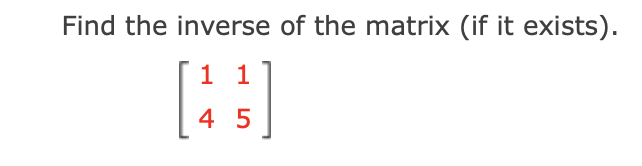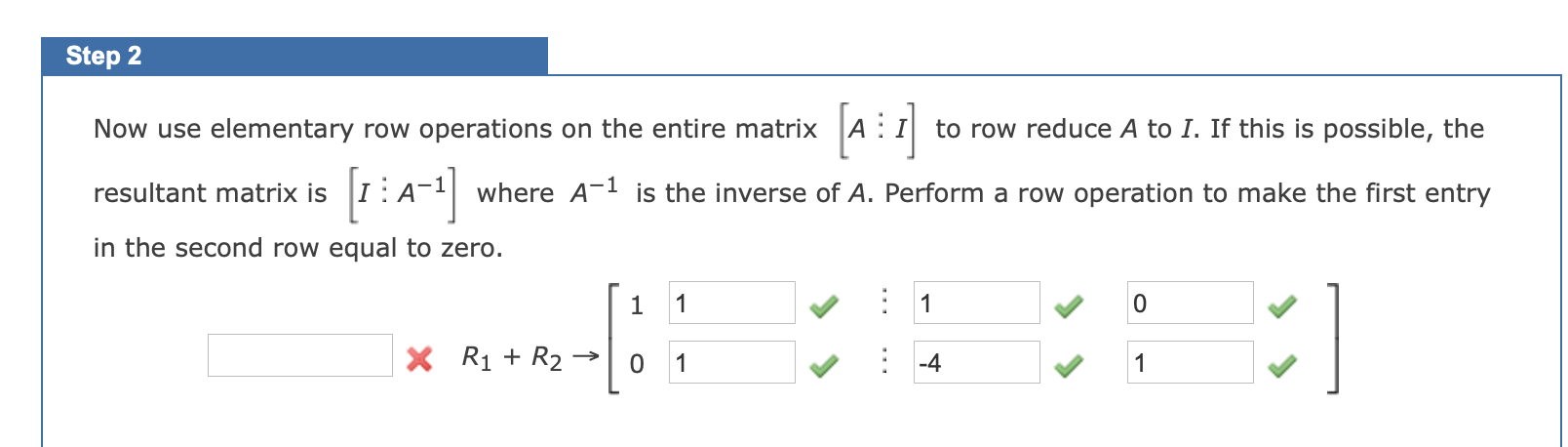# Question what goes in the box next to R1 please let me know Find the inverse of the matrix (if it exists). 1 1 4 5 Step 2 Now use elementary row operations on the entire matrix to row reduce A to I. If this is possible, the resultant matrix is A where A-1 is the inverse of A. Perform a row operation to make the first entry in the second row equal to zero. 1 : 1 0 X R1 + R2 0 1 : -4 1what goes in the box next to R1 please let me know

Transcribed Image Text: Find the inverse of the matrix (if it exists). 1 1 4 5 Step 2 Now use elementary row operations on the entire matrix to row reduce A to I. If this is possible, the resultant matrix is A where A-1 is the inverse of A. Perform a row operation to make the first entry in the second row equal to zero. 1 : 1 0 X R1 + R2 0 1 : -4 1
More
Transcribed Image Text: Find the inverse of the matrix (if it exists). 1 1 4 5 Step 2 Now use elementary row operations on the entire matrix to row reduce A to I. If this is possible, the resultant matrix is A where A-1 is the inverse of A. Perform a row operation to make the first entry in the second row equal to zero. 1 : 1 0 X R1 + R2 0 1 : -4 1